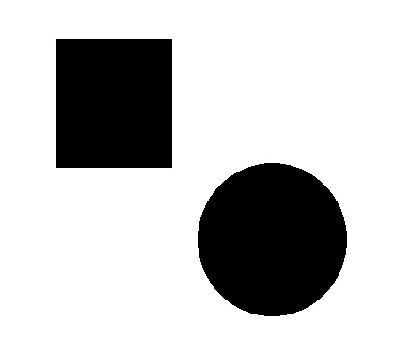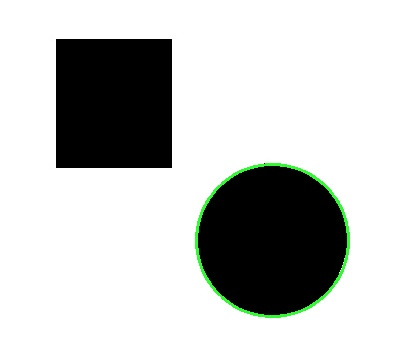• # Circle Detection

• Circle detection is the process of detecting and locating circular shapes in images, using OpenCV.

• In OpenCV, it is implemented with the HoughCircles() function.

• This function takes an image as input, and performs a series of steps to detect circles in the image.

In this tutorial, we’ll learn about how to detect circles in images using OpenCV. We’ll also share the code to detect circles from images.

# What is cv2.HoughCircles in OpenCV?

• It is a function in OpenCV used to detect circles in an image.

• It takes in an image and some parameters related to the circle detection, such as the minimum and maximum radius and threshold values.

InputCode:

``````import cv2
import numpy as np
gray = cv2.cvtColor(img, cv2.COLOR_BGR2GRAY)
blur = cv2.GaussianBlur(gray, (5, 5), 0)
if circles is not None:
circles = np.round(circles[0, :]).astype("int")
for (x, y, r) in circles:
cv2.circle(img, (x, y), r, (50, 250, 50), 2)
cv2.imshow('Image with Corners', img)
cv2.imwrite('dotted.jpg', img)
cv2.waitKey(0)
cv2.destroyAllWindows()``````
• First we imported required libraries.

• Then, we used cv2.imread() to read the image and cv2.cvtcolor() to convert the image into grayscale.

• Then, we applied the gaussian blur to smooth the image.

• Then, we used cv2.HoughCircles() with some parameters to detect the circles.

• Then, we used an if condition to check if any circles were detected in the image.

• If any circle was detected we used np.round() to round the detected circles. And we also used the cv2.circle() to draw circles over them.

• At last, we used cv2.imshow function to display the final result.

Result: